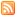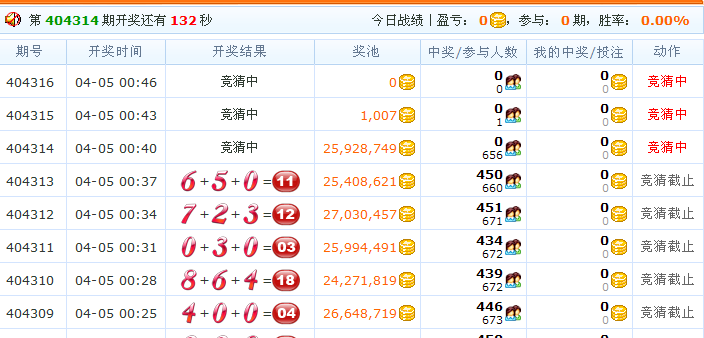25yicms利用ASP.NET(C#)+MSSQL技术全力打造功能最强大的营销型企业网站管理系统,企业做网站系统,做网站软件，提供div+css企业网站模板。RSS

## C#(asp.net)随机算法代码

C#(asp.net)随机算法应用在许多项目当中，我们详细讲解一下随机算法代码，以猜数字游戏为例。public int[] getRandomNum(int num, int min, int max,int b)
{
Random r = new Random(b * ((int)DateTime.Now.Ticks));
int[] a = new int[num];
int tmp = 0;
bool notRepeat;

for (int i = 0; i <= num - 1; )
{
tmp = r.Next(min, max); //随机取数
notRepeat = true;
if (notRepeat) a[i++] = tmp;
}
return a;

}

int[] a = getRandomNum(3, 0, 10,i);
model.Num1 = a;
model.Num2 = a;
model.Num3 = a;
model.NumSum = a + a + a;

作者: 网站设计@ 企业网站管理系统
原载: 25亿企业网站管理系统
版权所有。转载时必须以链接形式注明作者和原始出处及本声明。